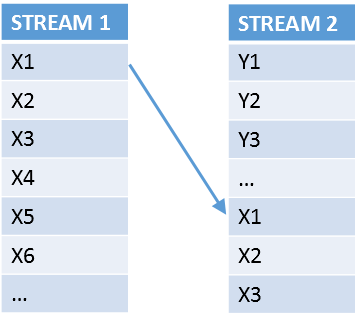Simulation studies require both randomness and reproducibility, two qualities that are sometimes at odds with each other. A Monte Carlo simulation might need to generate millions of random samples, where each sample contains dozens of continuous variables and many thousands of observations. In simulation studies, the researcher wants each sample to be statistically independent. For example, if you are drawing samples of size N=100 from a continuous distribution, it is not acceptable for the millionth sample to equal the first sample. Such a situation would bias the study. Similarly, it is not acceptable for half (or a fourth, or an eighth,...) of the numbers in the millionth sample to be the same as the numbers in the first sample.

The easiest way to ensure that each sample is statistically independent is to generate all samples from one high-quality random number stream. However, if the structure of your simulation requires that you use two or more streams, then SAS 9.4M5 provides several ways to produce random samples that are statistically independent. One way is to use one of the new Threefry random number generators. The other way is to use the new CALL STREAM subroutine.

### Independence and overlap in random number streams

I've previously written about the many random number generators (RNGs) in SAS. Recall that all RNGs produce uniform variates. When you call a RNG repeatedly, you are generating a stream of random numbers. A stream is the sequence of pseudorandom uniform values that is generated from a given seed value. (If you request a random value from a nonuniform distribution, such as normal or exponential, the software generates one or more uniform variates and then applies a mathematical transformation to produce a nonuniform variate.)In SAS, the modern RNGs are high quality and have a very long period. For all practical purposes, the samples that are generated from a single stream are independent. However, for the Mersenne twister and PCG RNGs, samples from different streams might not be independent. It might happen that the first sample for one stream is equal to (or has a large intersection with) the millionth sample from another seed, as shown in the image to the right. This phenomenon is known as overlap of streams.

The possibility that streams might overlap is important because some programmers use a macro loop to generate thousands of samples, where each sample is from a different stream. Not only is this technique inefficient, but the birthday problem in probability warns us that the probability that two streams overlap is larger than our intuition suggests.

The probability of overlapping streams is usually small, even for very large simulation studies. The "number of birthdays" equals the period of the RNG, which for Mersenne twister RNG is astronomically huge (≈ 106000). However, for the PCG RNG (which has a smaller period of "only" 264 ≈ 1019), the probability of an overlap could be nonnegligible.

Vigna (2009) estimates the probability of overlap when you use the first L variates in each of n streams from a RNG that has period P. Assuming that nL/P ≪ 1, the probability is approximately n2L/P. When P = 1019 and you generate n = 10,000 different streams, each containing L = 1,000,000 variates, the probability of an overlap is approximately 10-5.

Fortunately, SAS makes it it is easy to ensure the independence of random samples:

• As shown in Simulating Data with SAS and in many blog posts, you can almost always use a single stream to generate all the random samples.
• You can use one of the Threefry generators, TF2 or TF4. For a Threefry generator, two streams with different seed values never overlap! Similarly, streams for the hardware-based RDRAND generator never overlap.
• You can use the CALL STREAM subroutine to set a key value for the PCG algorithm. For the PCG RNG, streams that have the same seed value but different key values never overlap.

In my next blog post, I will demonstrate how to use the STREAM subroutine in the SAS DATA step to generate independent streams. The technique can be useful when you are running a simulation that requires several DATA steps, each of which generates random numbers.

Share